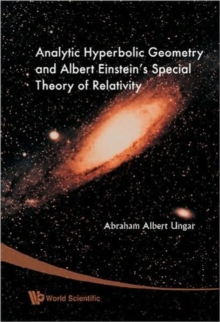Supporting your high street Find out how »
• My AccountAnalytic Hyperbolic Geometry And Albert Einstein's Special Theory Of Relativity Hardback

Description

This book presents a powerful way to study Einstein's special theory of relativity and its underlying hyperbolic geometry in which analogies with classical results form the right tool.

It introduces the notion of vectors into analytic hyperbolic geometry, where they are called gyrovectors.Newtonian velocity addition is the common vector addition, which is both commutative and associative.

The resulting vector spaces, in turn, form the algebraic setting for the standard model of Euclidean geometry.

In full analogy, Einsteinian velocity addition is a gyrovector addition, which is both gyrocommutative and gyroassociative.

The resulting gyrovector spaces, in turn, form the algebraic setting for the Beltrami-Klein ball model of the hyperbolic geometry of Bolyai and Lobachevsky.

Similarly, Moebius addition gives rise to gyrovector spaces that form the algebraic setting for the Poincare ball model of hyperbolic geometry.In full analogy with classical results, the book presents a novel relativistic interpretation of stellar aberration in terms of relativistic gyrotrigonometry and gyrovector addition.

Furthermore, the book presents, for the first time, the relativistic center of mass of an isolated system of noninteracting particles that coincided at some initial time t = 0.

The novel relativistic resultant mass of the system, concentrated at the relativistic center of mass, dictates the validity of the dark matter and the dark energy that were introduced by cosmologists as ad hoc postulates to explain cosmological observations about missing gravitational force and late-time cosmic accelerated expansion.The discovery of the relativistic center of mass in this book thus demonstrates once again the usefulness of the study of Einstein's special theory of relativity in terms of its underlying analytic hyperbolic geometry.

Information

• Format: Hardback
• Pages: 648 pages
• Publisher: World Scientific Publishing Co Pte Ltd
• Publication Date:
• Category: Relativity physics
• ISBN: 9789812772299

£149.00

£128.39

on all orders

Pick up orders

from local bookshops